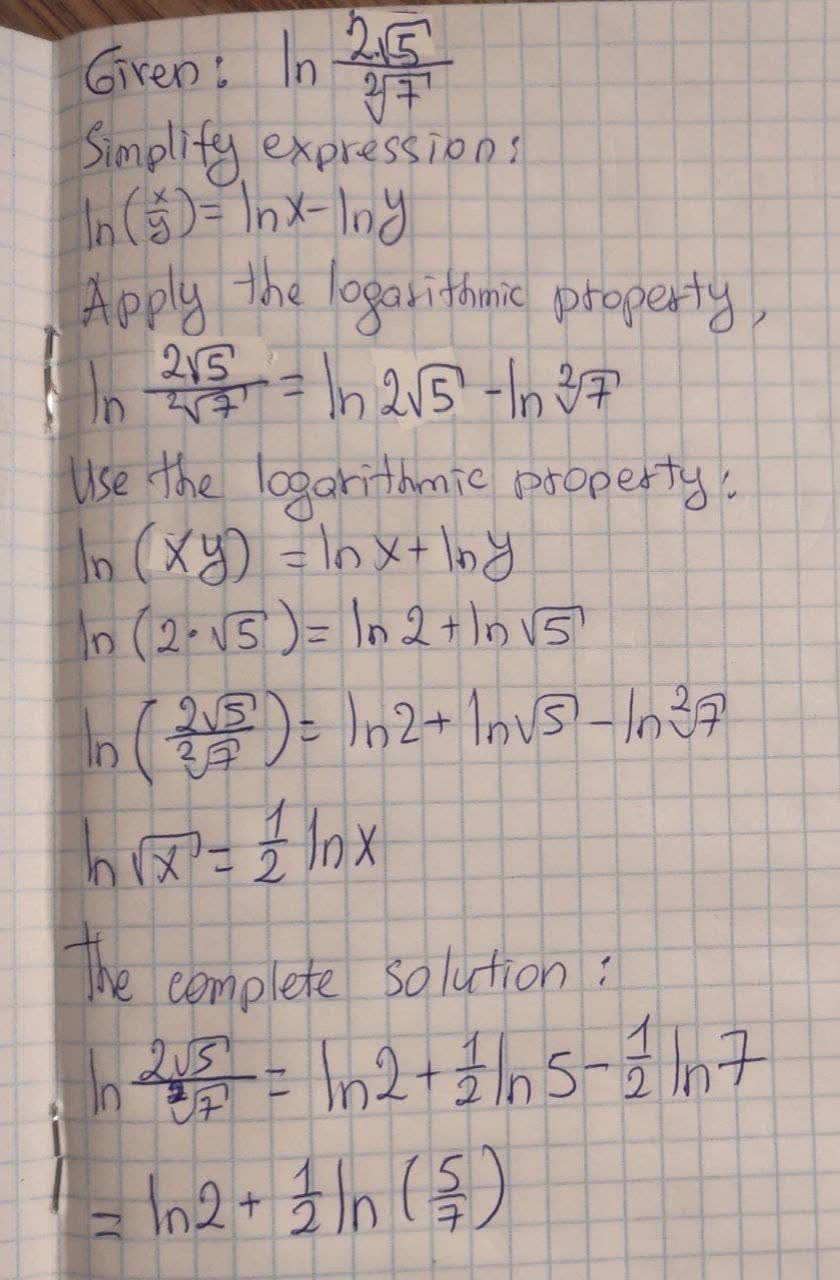# Use the properties of logarithms to write expression as aremolatg 2021-08-21 Answered

Use the properties of logarithms to write expression as a sum, difference, or product of simpler logarithms.
$$\ln \frac{2\sqrt{5}}{\sqrt{7}}$$

• Questions are typically answered in as fast as 30 minutes

### Plainmath recommends

• Get a detailed answer even on the hardest topics.
• Ask an expert for a step-by-step guidance to learn to do it yourself.Sadie Eaton
The solution is written below